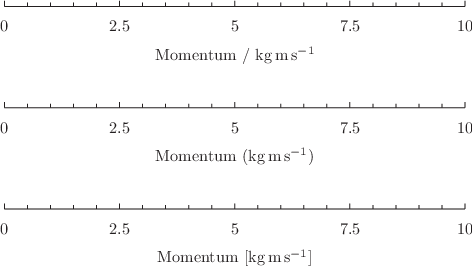## 8.8.3 Plotting quantities with physical units

When data with non-dimensionless physical units are plotted against an axis, for example using any of the statements

```plot [0:10] x*unit(m)
plot [0:10] x using 1:\$2*unit(m)
plot [0*unit(m):1*unit(m)] x**2

set unit angle nodimensionless ; plot [0:1] asin(x)
```

the axis is set to share the particular physical dimensions of that unit, and thereafter no data with any other physical dimensions may be plotted against that axis. When the axis comes to be drawn, Pyxplot makes a decision about which physical unit should be used to label the axis. For example, in the default SI system and with no preferred unit of length set, axes with units of length might be displayed in millimeters, meters or kilometers depending on their scales.

The chosen unit is indicated in one of three styles in the axis label, selected using the set axisunitstyle command:

```set axisunitstyle ratio
set axisunitstyle bracketed
set axisunitstyle squarebracketed
```

The effect of these three options, respectively, is shown below for an axis with units of momentum. In each case, the axis label was set simply using

```set xlabel "Momentum"
```

and the subsequent text was appended automatically by Pyxplot:When the set xformat command is used (see Section 8.8.8), no indication of the units associated with axes are appended to axis labels, as the set xformat command can be used to hard-code this information. The user must include this information in the axis label manually if it is needed.